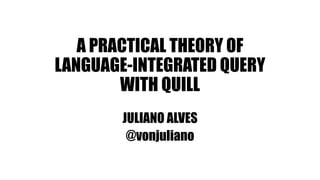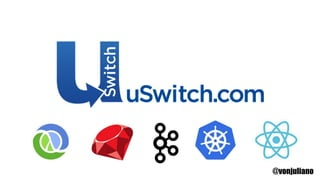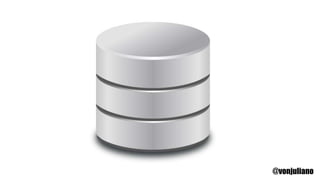Seu SlideShare está sendo baixado. ×

# A Practical Theory of Language-Integrated Query with Quill

Anúncio
Anúncio
Anúncio
Anúncio
Anúncio
Anúncio
Anúncio
Anúncio
Anúncio
Anúncio
AnúncioCarregando em…3
×

1 de 32 Anúncio

# A Practical Theory of Language-Integrated Query with Quill

Quill is a compile-time language integrated query library with a powerful query compilation mechanism based on the paper "A Practical Theory of Language-Integrated Query". This talk will give an overview of the query compilation, present the Quoted Domain Specific Language (QDSL) approach, and give a quick view of Quill's functionality and internals.

Quill is a compile-time language integrated query library with a powerful query compilation mechanism based on the paper "A Practical Theory of Language-Integrated Query". This talk will give an overview of the query compilation, present the Quoted Domain Specific Language (QDSL) approach, and give a quick view of Quill's functionality and internals.

Anúncio
Anúncio

### A Practical Theory of Language-Integrated Query with Quill

1. 1. A PRACTICAL THEORY OF LANGUAGE-INTEGRATED QUERY WITH QUILL JULIANO ALVES @vonjuliano
2. 2. @vonjuliano
3. 3. @vonjuliano
4. 4. @flaviowbrasil https://github.com/getquill/quill
5. 5. A PRACTICAL THEORY OF LANGUAGE- INTEGRATED QUERY @vonjuliano http://homepages.inf.ed.ac.uk/slindley/papers/practical-theory-of-linq.pdf
6. 6. SCYLLA – FAILURE CHARYBDIS – AVALANCHE @vonjuliano
7. 7. @vonjuliano
8. 8. @vonjuliano
9. 9. RESTRICTIONS SINGLE DB RETURNS A FLAT RELATION TYPE ONLY KNOWN OPERATIONS @vonjuliano
10. 10. SELECT w.NAME, w.age − m.age, FROM couples c, people w, people m WHERE c.her = w.NAME AND c.him = m.NAME AND w.age > m.age @vonjuliano
11. 11. quote { for { c <- couples w <- people m <- people if (c.her == w.name && c.him == m.name && w.age > m.age) } yield { (w.name, w.age - m.age) } } @vonjuliano
12. 12. quote { for { c <- couples w <- people m <- people if (c.her == w.name && c.him == m.name && w.age > m.age) } yield { (w.name, w.age - m.age) } } @vonjuliano
13. 13. FlatMap(Entity(Couple,None,List()),Ident(c),FlatMap(Entity(Per son,None,List()),Ident(w),Map(Filter(Entity(Person,None,List()), Ident(m),BinaryOperation(BinaryOperation(BinaryOperation(Pr operty(Ident(c),her),==,Property(Ident(w),name)),&&,BinaryOp eration(Property(Ident(c),him),==,Property(Ident(m),name))),& &,BinaryOperation(Property(Ident(w),age),>,Property(Ident(m), age)))),Ident(m),Tuple(List(Property(Ident(w),name), BinaryOperation(Property(Ident(w),age),- ,Property(Ident(m),age))))))) @vonjuliano
14. 14. @vonjuliano
15. 15. FlatMap(Entity(Couple,None,List()),Ident(c),FlatMap(Entity(Pe rson,None,List()),Ident(w),Map(Filter(Entity(Person,None,List( )),Ident(m),BinaryOperation(BinaryOperation(BinaryOperation (Property(Ident(c),her),==,Property(Ident(w),name)),&&,Binary Operation(Property(Ident(c),him),==,Property(Ident(m),name)) ),&&,BinaryOperation(Property(Ident(w),age),>,Property(Ident (m),age)))),Ident(m),Tuple(List(Property(Ident(w),name), BinaryOperation(Property(Ident(w),age),- ,Property(Ident(m),age))))))) SELECT w.NAME, w.age − m.age, FROM couples c, people w, people m WHERE c.her = w.NAME AND c.him = m.NAME AND w.age > m.age + = @vonjuliano
16. 16. ABSTRACTING OVER VALUES @vonjuliano
17. 17. val range = quote { (a: Int, b: Int) => for { u <- query[Person] if (a <= u.age && u.age < b) } yield { u } } db.run(range(10, 20)) @vonjuliano
18. 18. val range = quote { (a: Int, b: Int) => for { u <- query[Person] if (a <= u.age && u.age < b) } yield { u } } db.run(range(10, 20)) @vonjuliano
19. 19. val range = quote { (a: Int, b: Int) => for { u <- query[Person] if (a <= u.age && u.age < b) } yield { u } } db.run(range)(min, max) @vonjuliano
20. 20. ABSTRACTING OVER A PREDICATE
21. 21. val satisfies = quote { (p: Int => Boolean) => for { u <- query[Person] if (p(u.age)) } yield { u } } db.run(satisfies((x: Int) => 20 <= x)) @vonjuliano
22. 22. val satisfies = quote { (p: Int => Boolean) => for { u <- query[Person] if (p(u.age)) } yield { u } } db.run(satisfies((x: Int) => 20 <= x)) @vonjuliano
23. 23. COMPOSING QUERIES @vonjuliano
24. 24. val ageFromName = quote { (s: String) => for { u <- query[Person] if (s == u.name) } yield { u.age } } quote { (s: String, t: String) => for { a <- ageFromName(s) b <- ageFromName(t) r <- range(a, b) } yield { r } } @vonjuliano
25. 25. val ageFromName = quote { (s: String) => for { u <- query[Person] if (s == u.name) } yield { u.age } } quote { (s: String, t: String) => for { a <- ageFromName(s) b <- ageFromName(t) r <- range(a, b) } yield { r } } @vonjuliano
26. 26. DYNAMICALLY GENERATED QUERIES @vonjuliano
27. 27. sealed trait Predicate case class Above(i: Int) extends Predicate case class Below(i: Int) extends Predicate case class And(a: Predicate, b: Predicate) extends Predicate case class Or(a: Predicate, b: Predicate) extends Predicate case class Not(p: Predicate) extends Predicate def eval(t: Predicate): Quoted[Int => Boolean] = t match { case Above(n) => quote((x: Int) => x > lift(n)) case Below(n) => quote((x: Int) => x < lift(n)) case And(t1, t2) => quote((x: Int) => eval(t1)(x) && eval(t2)(x)) case Or(t1, t2) => quote((x: Int) => eval(t1)(x) || eval(t2)(x)) case Not(t0) => quote((x: Int) => !eval(t0)(x)) } @vonjuliano
28. 28. sealed trait Predicate case class Above(i: Int) extends Predicate case class Below(i: Int) extends Predicate case class And(a: Predicate, b: Predicate) extends Predicate case class Or(a: Predicate, b: Predicate) extends Predicate case class Not(p: Predicate) extends Predicate def eval(t: Predicate): Quoted[Int => Boolean] = t match { case Above(n) => quote((x: Int) => x > lift(n)) case Below(n) => quote((x: Int) => x < lift(n)) case And(t1, t2) => quote((x: Int) => eval(t1)(x) && eval(t2)(x)) case Or(t1, t2) => quote((x: Int) => eval(t1)(x) || eval(t2)(x)) case Not(t0) => quote((x: Int) => !eval(t0)(x)) } @vonjuliano
29. 29. EXAMPLE @vonjuliano
30. 30. @vonjuliano
31. 31. A PRACTICAL THEORY OF LANGUAGE-INTEGRATED QUERY WITH QUILL JULIANO ALVES @vonjuliano THANK YOU!
32. 32. Q U E S T I O N S ? @vonjuliano

### Notas do Editor

• Compile-time Language Integrated Query for Scala
Boilerplate-free mapping: The database schema is mapped using simple case classes
Quoted DSL: Quill parses each quoted block of code (quotation) at compile time and translates them to an internal Abstract Syntax Tree (AST)
Compile-time query generation: The ctx.run call reads the quotation's AST and translates it to the target language at compile time
Compile-time query validation: (optional) the query is verified against the database at compile time and the compilation fails if it is not valid
• Failure and avalanche
• each query in the host language generate exactly one SQL query
• Failure and avalanche